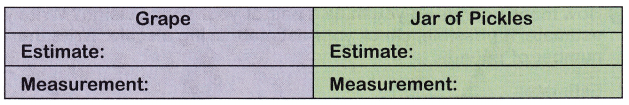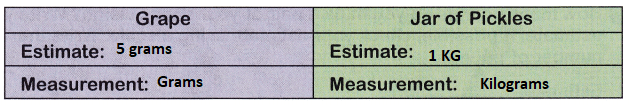# McGraw Hill Math Grade 3 Chapter 9 Lesson 6 Answer Key Mass in Grams and Kilograms

## McGraw-Hill Math Grade 3 Answer Key Chapter 9 Lesson 6 Mass in Grams and Kilograms

Estimate

Place a check mark beside the better estimate of each object’s mass.

Question 1.__________ 28 g
__________ 1 kg
The estimated weight of the pencil is 28 grams.

Question 2.__________ 30 kg
__________ 30 g
The estimated weight of monkey is 30 kg.

Question 3.__________ 10 kg
__________ 12 g
The estimated weight of the strawberry is 12 grams.

Question 4.__________ 5 g
__________ 1 kg